##### Statistics Essentials For Dummies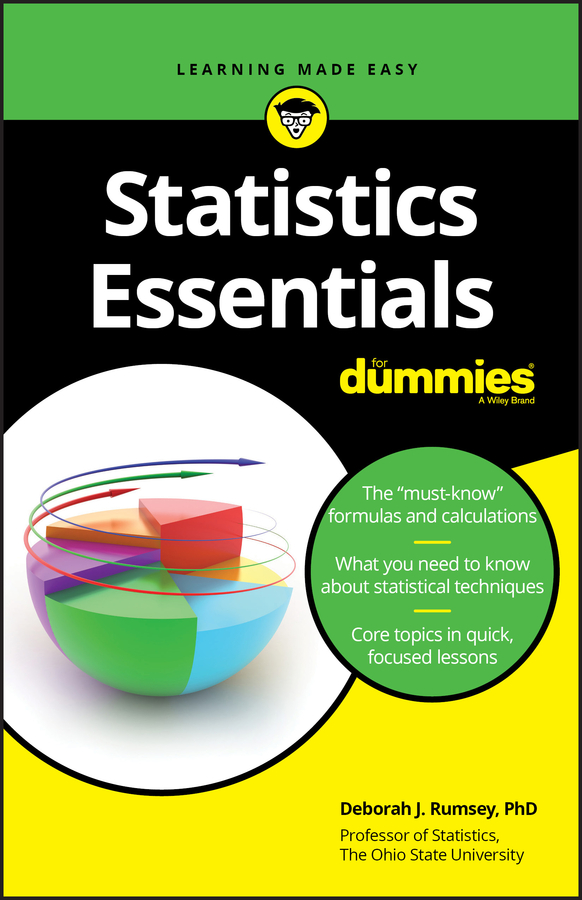If you focus on a closed interval along a continuous function, you can find the absolute extrema in that interval in one of three possible places: at a peak or valley or an edge(s) of the interval.

In this case, "absolute extrema" is just a fancy way of saying the single highest point and single lowest point in the interval.

Keep in mind that you could have, say, two peaks at the same height so there'd be a tie for the absolute max; but there would still be exactly one y value that's the absolute maximum value on the interval.

The following practice questions offer two instances where you have to find absolute extrema on an interval.

## Practice questions

1. Find the absolute extrema of f(x) = sin x + cos x on the interval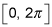2. Find the absolute extrema of g(x) = 2x3 – 3x2 – 5 on the interval [–0.5, 0.5].

1. The absolute max is at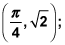the absolute min is atThis is illustrated in the following figure.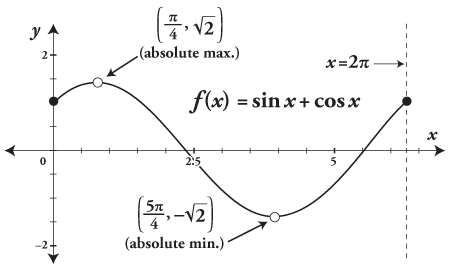You start by finding critical numbers.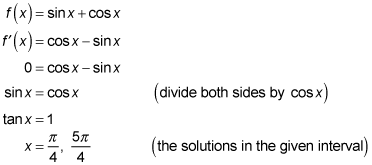The derivative is never undefined, so these are the only critical numbers.

Note that if you divide both sides of an equation by something that can equal zero at one or more x values (like you do here when dividing both sides by cos x), you may miss one or more solutions. You have to check whether any of those x values is a solution. In this problem, cos x = 0 at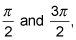and it's easy to check (as shown above) that sin x does not equal cos x at either of those values, so there's no problem here. But if sin x did equal cos x at either of those values, you'd have one or two more solutions and one or two more critical numbers. (Note that you have to check any such values in the line of the solution above where you do the dividing).

You can now evaluate the function at the critical numbers.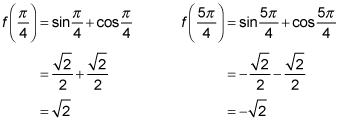Now evaluate the function at the endpoints of the interval.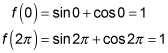The largest of the four answers is the absolute max; the smallest is the absolute min.

The absolute max is at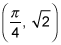The absolute min is at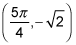2. The absolute min is at (–0.5, –6); the absolute max is at (0, –5).To find these numbers, you start by finding critical numbers.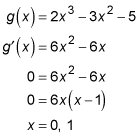x = 1is neglected because it's outside the given interval; x = 0 is your only critical number.

Now you evaluate the function at x = 0.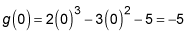Time to do the endpoint thing.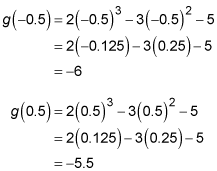Pick the smallest and largest answers.

The absolute min is at the left endpoint, (–0.5, –6). The absolute max is smack dab in the middle, (0, –5).